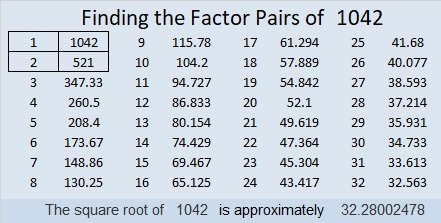# 1042 and Level 6

I’ve already published the two level 5 puzzles that are in this week’s set of puzzles. If going from a level 4 puzzle to a level 6 puzzle is too big of a jump for you, then try either one of those two level 5 puzzles first. You can find them as well as this puzzle in the link below the puzzle.Print the puzzles or type the solution in this excel file: 10-factors-1035-1043

Here are a few facts about the number 1042:

• 1042 is a composite number.
• Prime factorization: 1042 = 2 × 521
• The exponents in the prime factorization are 1 and 1. Adding one to each and multiplying we get (1 + 1)(1 + 1) = 2 × 2 = 4. Therefore 1042 has exactly 4 factors.
• Factors of 1042: 1, 2, 521, 1042
• Factor pairs: 1042 = 1 × 1042 or 2 × 521
• 1042 has no square factors that allow its square root to be simplified. √1042 ≈ 32.2800231²  + 9² = 1042

1042 is the hypotenuse of a Pythagorean triple:
558-880-1042 calculated from 2(31)(9), 31²  – 9², 31²  + 9²

1042 is also a palindrome in a couple of bases:
It’s 868 in BASE 11 because 8(121) + 6(11) + 8(1) = 1042, and
2C2 in BASE 20 (C is 12 in base 10) because 2(400) + 10(20) + 2(1) = 1042

This site uses Akismet to reduce spam. Learn how your comment data is processed.